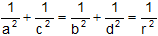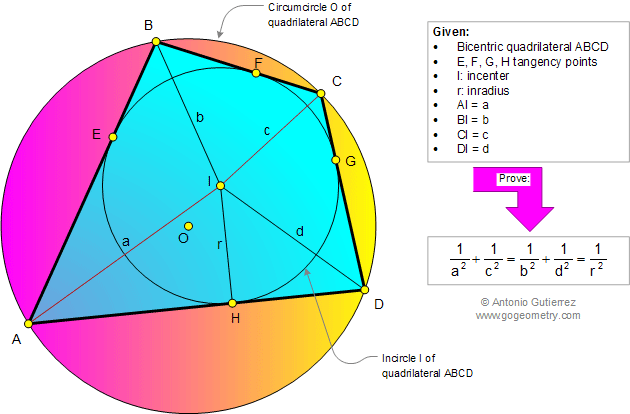# Online Geometry Problem 909: Bicentric Quadrilateral, Incircle, Circumcircle, Circumscribed, Inscribed, Tangent, Incenter, Inradius, Distance. Level: High School, College, Mathematics Education

< PREVIOUS PROBLEM  |  NEXT PROBLEM >

 In a bicentric quadrilateral ABCD, I is the incenter , O the circumcenter, and r the inradius, If AI = a, BI = b, CI =c, and DI = d, prove that. A bicentric quadrilateral ABCD is a convex quadrilateral that has both an incircle I and a circumcircle O.Home | Search | Geometry | Problems | All Problems | Open Problems | Visual Index | 10 Problems | Problems Art Gallery |  Art | 901-910 | Quadrilaterals | Cyclic Quadrilateral | Tangential or circumscribed quadrilateral | Circle Tangent Line | Solution / comment | by Antonio Gutierrez